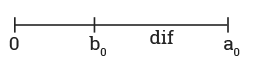# An Example of Swap Without Temp

Here is a solution to the problem of swap without temp. Now a similar problem that a student may have can be solved as follows in our example. If our sample has made a good impression on you then you should consider getting help from AssignmentShark.com. Our expert will follow all of your requirements and academic standards while dealing with your order. Next, we should mention that we are available 24/7, so you can get our help any time you need.

The purpose of our service is to provide customers with qualified technical help with their homework. Even the most difficult task can be solved by our expert. Many students have already used our service and remain satisfied. Feedback that they have left can be easily found on our site. So, using our service, you can get a lot of benefits. It is clear that our samples can help you as well, so read the presented one to understand what we are talking about.

Swap the Values of Variables Without Use of the Temporary Variables

This is a classic problem. It is usually offered in interviews, and it is quite simple. Assume x0 is the original value of x, and y0 is the original value of y. Assume dif as a difference between x and y (x – b). Let’s show the relative position of all of these values on the real axis in the case when x > y.Assign x the value of dif. If we add the value of y and dif, we will get x0 and save the result to y. Now we have y = x0 and x = dif. Now all we have to do is assign y the value of x0 – dif, which is equal to y – x.

The following code implements this algorithm:

```public static void swap(int x, int y) {
//for example, let x = 10, y = 6;
x = x - y; // x = 10 - 4 = 6
y = x + y; // y = 6 + 4 = 10
x = y - x; // x = 10 - 6

System.out.println("x: " + x);
System.out.println("y: " + y);
}
```

We can also solve this problem using a bit manipulation. This approach will allow us to work with different types of data, not just integers.

```public static void swap(int x, int y) {
//for example, x = 101 (binary), y = 110
x = x ^ y; // x = 101^110 = 011
y = x ^ y; // y = 011^110 = 101
x = x ^ y; // x = 011^101 = 110

System.out.println("x: " + x);
System.out.println("y: " + y);
}
```

This code uses the operation XOR. The easiest way to understand the code is to look at the two bits a and b. Let’s denote the initial values as a, a0, and b0.
If we will be able to swap a couple of bits, the algorithm will work correctly. Let’s look at the work of the algorithm step by step:

1. a = a0 ^ b0 (0 if a0 = b0, 1 if a0 ! = b0)
2. b = a ^ b0 (equal to the value of a0)
3. a = a ^ b (equal to b0)

In line 1 we perform a = a0^b0 operation, which will be equal to 0 if a0 = b0, and equal to 1 if a0 != b0.

In line 2 we perform the b = a ^ b0 operation. Let’s analyze both possible values of a. Since we want to swap the values of a and b, we want to get 0 as a result:

• a = 0: in this case a0 = b0 because we want to return a0 and b0. Applying XOR for any value will return the original value, so the result of this operation will be b0 (or a0).
• a = 1: in this case a0 != b0. As a result, we get the inversion of a0. This is exactly what we want because a0 != b0.

at the moment is equal to a0; so, in fact, we do a^a0.

• a = 0: Since a0 = b0, we want to return a0 or b0. Performing 0^a0 we will return a0(b0).
• a = 1: We perform 1^a0. As a result, we will get an inversion of a0, which is exactly what we need, because a0 != b0.

Now we should assign a the value of b0, and assign b the value of a0. Now we are sure that our algorithm reverses each bit correctly, which means that the result is correct.